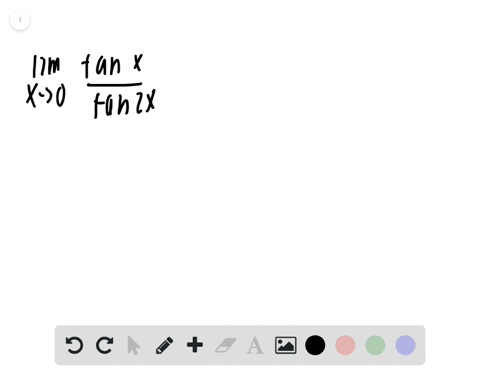Enroll in one of our FREE online STEM bootcamps. Join today and start acing your classes!View Bootcamps## Discussion

You must be signed in to discuss.

## Video Transcript

Okay, So we were asked to create a table with picked always from our left hand side. We'll start with Ecstasy Equity negative 0.1, and then we plug in Exeter. Gets to make it a 0.1 and two signs two times for you to put one over. Negative one to get our function is approximately 1.98669 excuse Actually, could be a negative 0.1 Then our function is approximately 1.999 And then if X equals negative points, so no one, our function is approximately 1.9999 if X is equal to the value from a right hand side of Joe. So if I choose 0.1 our function is approximately 1.9999 If X is equal to point, there are one. Our function is approximately 1.99987 If you know I'm here right here would be where X is equal to zero in. So as we're approaching from the last time, right, we're getting 0.999 and we're working from the right hand side. We're getting 1.999 He'll be around that, uh, the limit as expertise, ill of our function is approaching. You